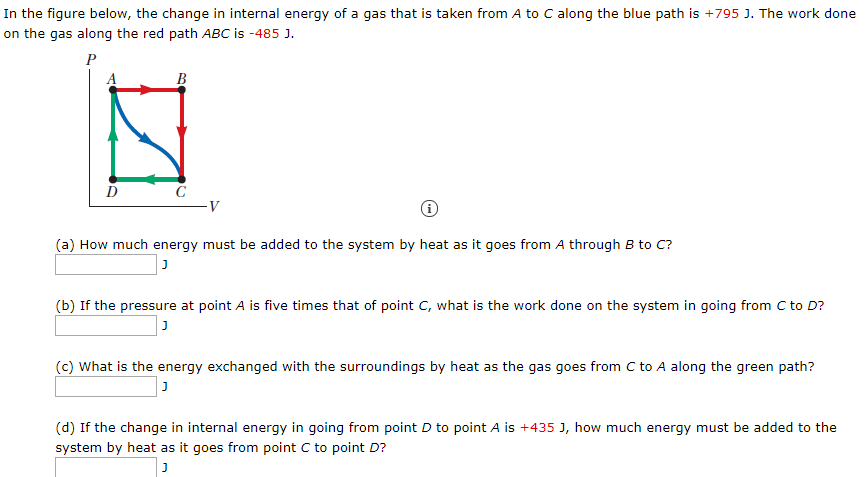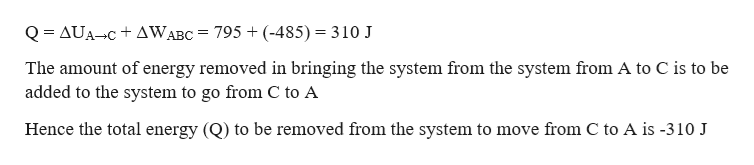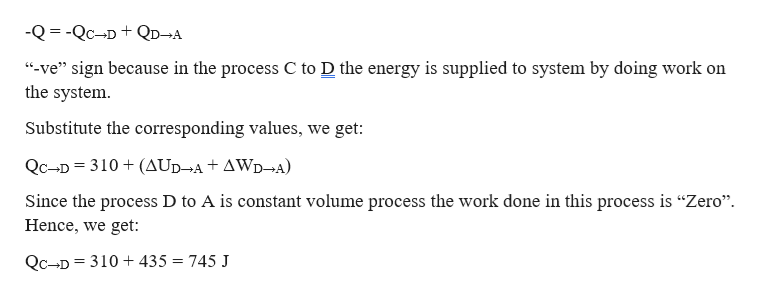# In the figure below, the change in internal energy of a gas that is taken from A to C along the blue path is +795 J. The work doneon the gas along the red path ABC is -485 J.B(a) How much energy must be added to the system by heat as it goes from A through B to C?(b) If the pressure at point A is five times that of point C, what is the work done on the system in going from C to D?(c) What is the energy exchanged with the surroundings by heat as the gas goes from C to A along the green path?(d) If the change in internal energy in going from point D to point A is +435 J, how much energy must be added to thesystem by heat as it goes from point C to point D?

Question
58 viewshelp_outlineImage TranscriptioncloseIn the figure below, the change in internal energy of a gas that is taken from A to C along the blue path is +795 J. The work done on the gas along the red path ABC is -485 J. B (a) How much energy must be added to the system by heat as it goes from A through B to C? (b) If the pressure at point A is five times that of point C, what is the work done on the system in going from C to D? (c) What is the energy exchanged with the surroundings by heat as the gas goes from C to A along the green path? (d) If the change in internal energy in going from point D to point A is +435 J, how much energy must be added to the system by heat as it goes from point C to point D? fullscreen
check_circle

Step 1

Given information:

Change in internal energy as the system moves from point A to C (ΔUA→C) = +795 J

The work done on the gas along ABC is (ΔWABC) = -485 J

The internal energy change as the system moves from D to A (ΔUD→A) = -485 J

Step 2

The total energy added to the system while taking it from point A to C through the path ABC is given by:help_outlineImage TranscriptioncloseQ = AUA-C + AWABC = 795 + (-485) = 310 J The amount of energy removed in bringing the system from the system from A to C is to be added to the system to go from C to A Hence the total energy (Q) to be removed from the system to move from C to A is -310 J fullscreen
Step 3

It is given as sum of the energies supplied in taking system through the...help_outlineImage Transcriptionclose-Q = -Qc-D +QD¬A "-ve" sign because in the process C to D the energy is supplied to system by doing work on the system. Substitute the corresponding values, we get: Qc-D = 310 + (AUD¬A + AWD¬A) Since the process D to A is constant volume process the work done in this process is "Zero". Hence, we get: Qc-D = 310 + 435 = 745 J fullscreen

### Want to see the full answer?

See Solution

#### Want to see this answer and more?

Solutions are written by subject experts who are available 24/7. Questions are typically answered within 1 hour.*

See Solution
*Response times may vary by subject and question.
Tagged in
SciencePhysics

### Thermodynamics